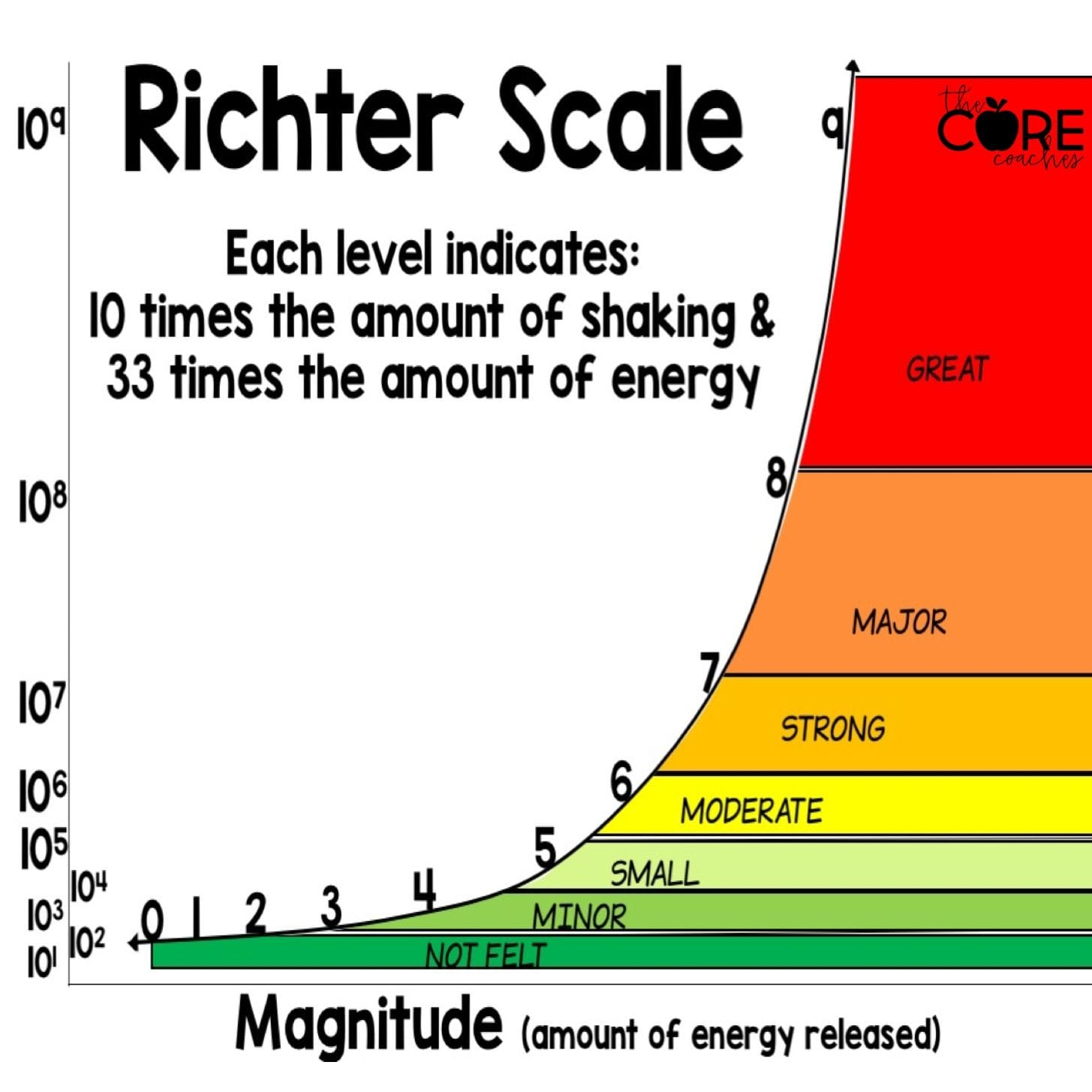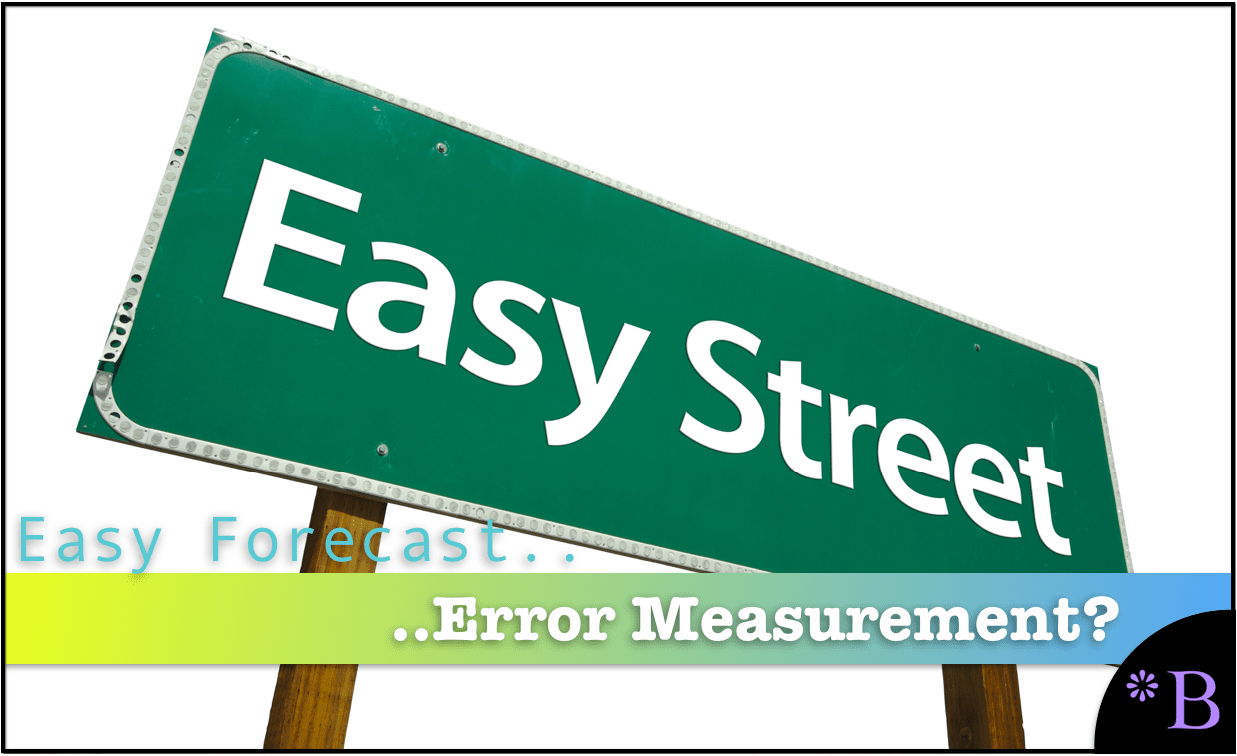# How MAD is Calculated for Forecast Error Measurement

Last Updated on April 14, 2021 by

## Executive Summary

• MAD is a universally accepted forecast error measurement.
• MAD is generally low in effectiveness in providing feedback to improve the forecast.## Text Introduction (Skip if You Watched the Video)

MAD or Mean Absolute Deviation is one of the most common forecast error measurement in use. MAD is moderately easy to understand and relatively easy to calculate. However, when MAD is used, in most cases, the problems with MAD are not explained to the audience, which is an issue that also generalizes to the other primary forecast error measurement calculations. At Brightwork, we do not use MAD when we perform forecast analysis. In this article, you will learn about the issues with MAD that reduce the ability of a person or company to improve their forecast accuracy.

If you want to see our references for this article and other related Brightwork articles, visit this link.

How MAD is calculated is one of the most common questions we get.

1. Find the mean of the actuals.
2. Subtract the mean of the actuals from the forecast and use the absolute value.
3. Add all of the errors together.
4. Divide by the number of data points.

The formula is..

### = SUM(ABS(F – MA))/# of Data Points

Like MAPE, MAD uses absolute values, so one does not understand the bias. But because MAD is squared, the error is not proportional — meaning that larger errors become much larger as they are squared. Any error measurement that is not proportional makes it intuitively more difficult to understand than a proportional measure. How is, particularly, a nonforecasting focused person supposed to interpret non-proportional forecast errors? Our brain is designed to understand proportionality.For example, the Richter Scale is a logarithmic scale, which allows a relatively small number of values to represent large magnitudes in difference. However, it also means that humans cannot calculate the difference between different earthquake values.

## The Same Problem With All the Standard Forecast Error Measurements

How MAD is calculated is one of the most common questions we get. But the narrow question broadens out when one looks at the different dimensions of forecast error.

Due to its lack of proportionality, MAD was never a forecast error method before developing our own. However, in addition to this lack of proportionality, MAD shares the same problems with all standard forecast error measurements.

These include the following:

1. They are difficult to weigh. And any forecast error aggregated beyond the product location combination must be weighed to make any sense.
2. They make comparisons between different forecasting methods overly complicated.
3. They lack the context of the volume of the demand history or the price of the product being forecasted, meaning that the forecast errors must be provided with context through another formula.
4. They are difficult to explain, making them less intuitive than a different approach.

## Why Do the Standard Forecast Error Calculations Make Forecast Improvement So Complicated and Difficult?

It is important to understand forecasting error, but the problem is that the standard forecast error calculation methods do not provide this good understanding. In part, they don't let tell companies that forecast how to make improvements. If the standard forecast measurement calculations did, it would be far more straightforward and companies would have a far easier time performing forecast error measurement calculation.

## What the Forecast Error Calculation and System Should Be Able to Do

One would be able to for example:

1. Measure forecast error
2. Compare forecast error (For all the forecasts at the company)
3. To sort the product location combinations based on which product locations lost or gained forecast accuracy from other forecasts.
4. To be able to measure any forecast against the baseline statistical forecast.
5. To weigh the forecast error (so progress for the overall product database can be tracked)

##Getting to a Better Forecast Error Measurement Capability

A primary reason these things can not be accomplished with the standard forecast error measurements is that they are unnecessarily complicated, and forecasting applications that companies buy are focused on generating forecasts, not on measuring forecast error outside of one product location combination at a time. After observing ineffective and non-comparative forecast error measurements at so many companies, we developed, in part, a purpose-built forecast error application called the Brightwork Explorer to meet these requirements.

Few companies will ever use our Brightwork Explorer or have us use it for them. However, the lessons from the approach followed in requirements development for forecast error measurement are important for anyone who wants to improve forecast accuracy.